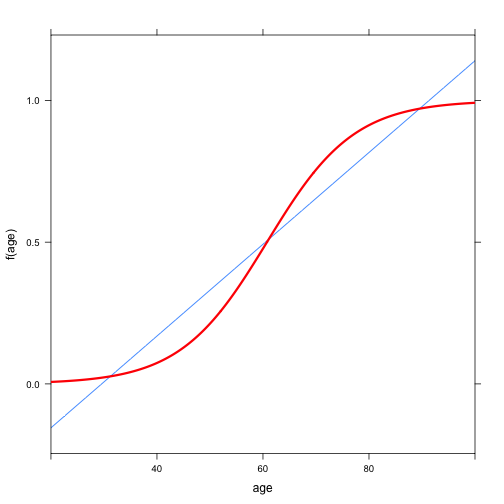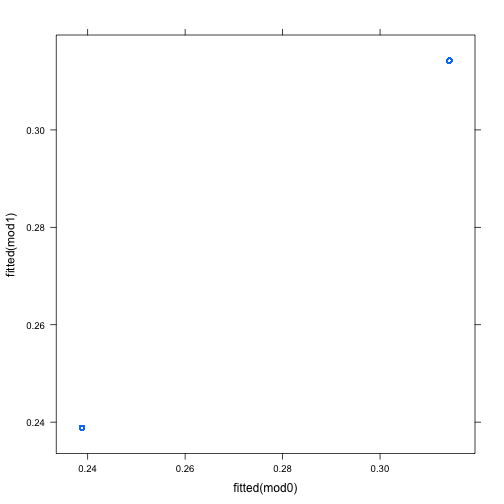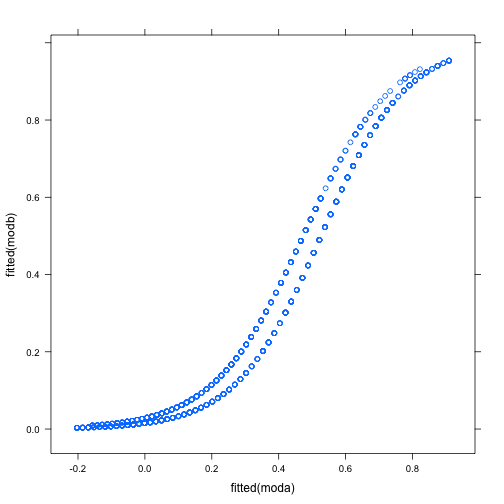# Stats 155 Class Notes 2012-11-26

### Review of Odds

An introduction to being a bookie.

Who is going to win the Superbowl? Solicit answers. Add “the Twins” to the list. Then go through the class and get people to lay a $1 or$2 bet on their choice.

For each choice, the odds are simply calculated: the amount on that choice divided by the amount on all the other choices.

If you win, you get your bet times the odds. (You also keep your original money.) If you lose, you pay up.

Show that I, the bookie, can't lose money — the payout in every case must be exactly equal to the amount paid by the people who lose.

By shaving the odds a bit, I can guarantee to make money. (At least, if I can collect from the losers.)

In practice, it works like this:

• You call me up and lay a bet.
• If you lose, I keep track of your debt to me. When this reaches too high a level, I threaten to break your legs.
• If you win, I pay you. Most likely, I just cancel your winnings from your already existing debt.

So, with a bit of seed capital, and some muscle for enforcement, I can make money.

An explanation resource on Dutch book

### Review of Logistic Regression

#### Fitting logistic models

• Constructing a yes/no variable with ==
• glm and the family="binomial"
• The summary report

#### Confounding, covariates, and Logistic Regression

The Whickham data.

This is drawn from a cohort study of nurses in Whickhamshire, England. Nurses were asked many questions about their lifestyle, diet, etc. Then they were revisited 20 years later. This small data set just records their age at the first interview, whether they identified themselves as smokers, and whether they were still alive at the 20 year follow-up.

w = fetchData("whickham.csv")

## Retrieving from http://www.mosaic-web.org/go/datasets/whickham.csv


First, let's look at the relationship between age and probability of being dead at the follow-up. We'll compare a straightforward linear model with a logistic regression model:

m = lm(outcome == "Dead" ~ age, data = w)
m2 = glm(outcome == "Dead" ~ age, data = w, family = "binomial")
f = makeFun(m)
f2 = makeFun(m2)
plotFun(f(age) ~ age, age.lim = c(20, 100))
plotFun(f2(age) ~ age, add = TRUE, col = "red", lwd = 3)Note that the models agree on the probability of death (before the 20 year follow-up) for women in their middle ages.

• At the extremes, the linear model is silly. It has probability outside the range 0 to 1.
• It doesn't work so well on the inside either, since the linear function can't be steeper in the center without being absurdly high near the edges.

#### Interpreting the coefficients

coef(summary(m))

##             Estimate Std. Error t value   Pr(>|t|)
## (Intercept) -0.47880  0.0277403  -17.26  2.576e-60
## age          0.01619  0.0005542   29.21 6.517e-145


The coefficient on age tells how the probability changes with an increase of one year in age. This simple linear model says that the change in probability is constant across the ages. (We asked it to say that: it's a linear model!)

• Linear Model: the probability of dying increases by 1.6 percentage points each year.
• Logistic Model: the odds of dying increases by 12% per year. That's not percentage points, but a multiplier of 0.12. In other words, the odds of dying increases exponentially.
• QUESTION: This means that the odds reaches $$\infty$$. What probability corresponds to an odds of $$\infty$$?

#### 95% confidence interval on the rate of odds increase

exp(log(0.122) + 2 * c(-1, 1) * 0.00694)

##  0.1203 0.1237


#### Smoking and Death

mod0 = lm(outcome == "Dead" ~ smoker, data = w)
mod1 = glm(outcome == "Dead" ~ smoker, data = w, family = "binomial")
coef(summary(mod0))

##             Estimate Std. Error t value  Pr(>|t|)
## (Intercept)  0.31421    0.01657  18.968 4.304e-71
## smokerYes   -0.07538    0.02489  -3.028 2.507e-03

coef(summary(mod1))

##             Estimate Std. Error z value  Pr(>|z|)
## (Intercept)  -0.7805    0.07962  -9.803 1.096e-22
## smokerYes    -0.3786    0.12566  -3.013 2.590e-03

xyplot(fitted(mod1) ~ fitted(mod0))Interpret the two models as probabilities. Translate the coefficients in the logistic model into probabilities in the second. They are essentially the same think. Both indicate that a smoker is significantly less likely to be dead.

Now include the covariate of age, perhaps with an interaction of age and smoker**:

moda = lm(outcome == "Dead" ~ smoker * age, data = w)
modb = glm(outcome == "Dead" ~ smoker * age, data = w, family = "binomial")
coef(summary(moda))

##                Estimate Std. Error t value   Pr(>|t|)
## (Intercept)   -0.506301  0.0359446 -14.086  4.625e-42
## smokerYes      0.082244  0.0574953   1.430  1.528e-01
## age            0.016848  0.0006887  24.466 3.492e-109
## smokerYes:age -0.002013  0.0011737  -1.715  8.659e-02

coef(summary(modb))

##               Estimate Std. Error z value  Pr(>|z|)
## (Intercept)   -8.16923   0.606600 -13.467 2.438e-41
## smokerYes      1.45784   0.837232   1.741 8.164e-02
## age            0.13323   0.009953  13.386 7.278e-41
## smokerYes:age -0.02223   0.014495  -1.534 1.250e-01

xyplot(fitted(modb) ~ fitted(moda))Notice how the linear model admits probabilities that are larger than 1.

#### Why not use a polynomial model with lm()

Polynomials go the wrong way: their tails head off to infinity. The use of odds and the logarithm essentially solve the problem of getting a function that behaves nicely.

### The odds ratio in use

A study of bicycle helmet use and the influence of state law.

Demonstration of logistic regression and odds ratios. “Effects of state helmet laws on bicycle helmet use by children and adolescents” Injury Prevention 2002, 9:42-46

Calculate the confidence interval on the odds ratio, e.g.

exp(0.7 + c(-1, 1) * 1.96 * 0.294)

##  1.132 3.583


### Introduction to R/Markdown

Activity**: Modeling Barry Bonds in 2001.

bonds = fetchData("bonds2001.csv")

## Retrieving from http://www.mosaic-web.org/go/datasets/bonds2001.csv


Source**: Journal of Statistics Education. Bonds data submitted by Jerome P. Reiter, Institute of Statistics and Decision Sciences, Duke University, Box 90251, Durham, NC 27708, jerry@stat.duke.edu

Student task**: Build a model of whether Bonds gets on base as a function of whatever other variables appear appropriate to you. Write it up in R/Markdown, including some nice graphic and an interpretation of your results.

Here's one model**:

mod1 = glm(reachesBase == 0 ~ outs + onFirst + onSecond + onThird + plateWithinGame,
data = bonds, family = "binomial")
summary(mod1)

##
## Call:
## glm(formula = reachesBase == 0 ~ outs + onFirst + onSecond +
##     onThird + plateWithinGame, family = "binomial", data = bonds)
##
## Deviance Residuals:
##    Min      1Q  Median      3Q     Max
## -1.491  -1.136  -0.803   1.153   1.669
##
## Coefficients:
##                 Estimate Std. Error z value Pr(>|z|)
## (Intercept)      -0.0796     0.2315   -0.34  0.73082
## outs             -0.1368     0.1016   -1.35  0.17842
## onFirst          -0.0984     0.1714   -0.57  0.56610
## onSecond         -0.7418     0.2246   -3.30  0.00096 ***
## onThird          -0.2954     0.2923   -1.01  0.31222
## plateWithinGame   0.1273     0.0589    2.16  0.03070 *
## ---
## Signif. codes:  0 '***' 0.001 '**' 0.01 '*' 0.05 '.' 0.1 ' ' 1
##
## (Dispersion parameter for binomial family taken to be 1)
##
##     Null deviance: 897.82  on 647  degrees of freedom
## Residual deviance: 877.21  on 642  degrees of freedom
## AIC: 889.2
##
## Number of Fisher Scoring iterations: 4


Evidently, Bonds was significantly more likely to get on base if there was another player on second base (onSecond), and taking into account his general downward trend over the course of a game (plateWithinGame)

Variables**:

• plateNo Plate appearance number.
• gameNo: Number of the game in the season.
• plateWithinGame: Number of the plate appearance within the game.
• inSF: Equals one for games in San Francisco and equals zero otherwise.
• onFirst: Equals one when there is a runner on first base when Bonds appears and equals zero otherwise.
• onSecond: Equals one when there is a runner on second base when Bonds appears and equals zero otherwise.
• onThird: Equals one when there is a runner on third base when Bonds appears and equals zero otherwise.
• outs: Number of outs in inning when Bonds appears.
• inning: Inning of plate appearance.
• runs: Number of runs scored by Giants in the inning after first pitch to Bonds.
• walk: Equals one if Bonds walks and equals zero otherwise.
• intentionalWalk: Equals one if Bonds walks intentionally and equals zero otherwise.
• reachesBase:
• Equals zero if Bonds does not reach base.
• Equals one if Bonds reaches first base on a single or error.
• Equals two if Bonds reaches second base on a double or error.
• Equals three if Bonds reaches third base on a triple or error.
• Equals four if Bonds hits a home run. Equals five if Bonds walks or is hit by a pitch.
• ERA: Opposing pitchers' career earned run average as of the end of the 2000 season.
• scoreGiants: Giants score just before first pitch to Bonds
• scoreOpponent: Opposing team's score just before first pitch to Bonds.